# アナログ回路設計コンサルタント　株式会社e-skett

## アナログ電子回路設計に関する技術コンサルティングのご用命は株式会社ｅ－ｓｋｅｔｔ

〒253-0012 茅ヶ崎市小和田三丁目８番-12-611号

How to use e-calcFree

## help

### How to use e-calcFree

Here is how to use the (e-calcFree(Ver1.1)) calculator app for iPhone in the iTunes App Store currently published.
(There is also a more powerful e-calcPro.)

This calculator can be used not only in scientific notation(e.g. 1E-6) but also using engineering multipliers(e.g. p,n,u,m,k,M).

This is a screen shot of the e-calcFree.。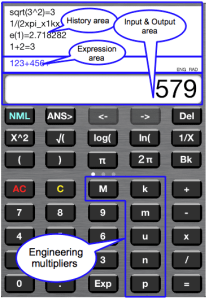Visible part is divided into three.
The bottom is the input and output display area,Similar to the general part of the calculator display.
On it, is the area formula to display the formula in the current calculation.
The top is a history area and to display the result of a calculation formula of the past.
There is a mode display area in the lower right corner of the area formula.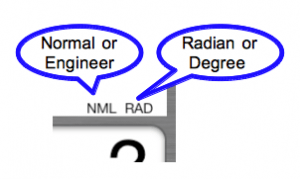You can use an electrical engineer commonly used engineering units in e-calcFree.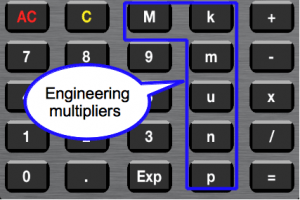Each button meaning,
p=1E-12,n=1E-9,u=1E-6,m=1E-3,k=1E3,M=1E6 .
Can be entered directly from the button is these six, the display is also used f = 1E-15, G = 1E9.
By pressing the  ENG(NML) button , you will be able to choose whether to use the exponential format display, whether to display using this auxiliary unit.
However,  in the case of the result  is less than 1E-16 or even more than 1E15  using the exponential form.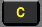is a clear button.s reset to 0 input and output display area.。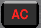is ALL Clear button. Clear input & output area  also Expression area.
Without pressing the AC button, after pressing the equal  button , so that the following calculations can continue.
Please press the AC button to do when things go wrong.
√ When calculating, please enter the order √ button, and then  numeric value.
We will be as follows: Press the button when calculating the √ 3, for example.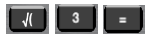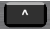This button is the exponentiation operator. We then used to calculate the x ^ y.
When you press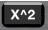button, after the number of the input area, the word ^ 2 is added.
not entered the right parenthesis, which is auto-completion.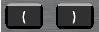Also, if when you press the = button, the number of right parentheses is not enough,  automatically insert the right parenthesis to the right with the necessary number of expressions.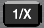When you press this button after entering 1 +2 +3, converted to expression 1 / (1 +2 +3) (only convert,  do not do the  calculation.)
Do not use this button, if you want the 1 +2 +1 / 3 Please enter formulas to reason.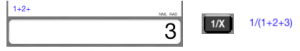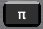This button will insert the word pi_ expression.  pi_ = 3.141593 … is the actual calculation.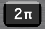This button is only to insert the word 2xpi_ expression. I think in the calculation, such as the cut-off frequency is convenient to use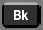This button will insert the word bk_ expression. This is the Boltzmann’s constant. Calculation time is converted to bk_ = 1.3807E-23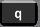button will insert the word q_ expression. This is the elementary charge. Calculation time is converted to q_ = 1.60218E-19.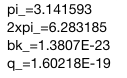You will be shown below when you swipe to the left to further function area.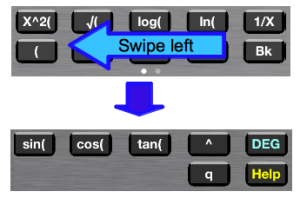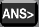This button copies the input and output display area the previous calculation results.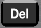This button is used to erase one character at the input and output display area.
Function or, in the case of the constant we clear the whole it.
When there are no more characters in the input and output display area, one character at a time to clear the character of area formulas.
You can continue to calculate the correct value and operation button after clearing.

Edit mode by tap the area formulas, which will change yellow.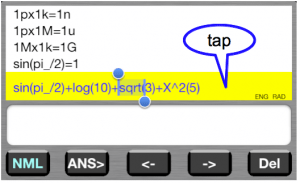his mode can be use cursor button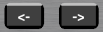you will be able to move the selected area using the cursor button.
Selected area will be erased when you press thisbutton.
When you press the function button and the calculation button and number button, the character is inserted to the right of the cursor.
Selection area extends to its length part of the functions and constants.
Press the cursor left button when the selected region further reaches the left end, the selected area will disappear, but if you type something at this time, characters will be inserted on the left side.
When you return to the normal mode by tapping the area formula again, you will be able to continue the calculation after editing.
It should be noted that, after even without entering the edit mode, press the = button, by pressing a button operation, if you want to calculate and add the expression to the result of the last, or you can add an expression to the formula of the previous can.

history of about four lines can be displayed in the iPhone, such as display of 3.5inch iPhone4.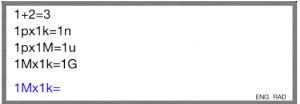iPhone5(4inch), it is possible to display the line about 9 .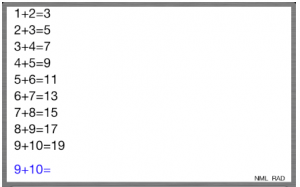There is also a more powerful e-calcPro.

e-calcPro’s Features

• Calculations History and reuse it
• Can use engineering multipliers,6 Special buttons
• Four user-defined constant
• Four interactive variable
• Three buttons can be insert formula
• interactive variable can have alias
• Functions: √,log,ln,sin,cos,tan,sinh,cosh,tanh,asin,acos,atan,asinh,acosh,atanh,e^x
• Built-in Constants:π,bk,q,e0 and Operator　^
• Autocomplete parentheses
• ON/OFF function key click sound
• Mail transmission function of the calculation history